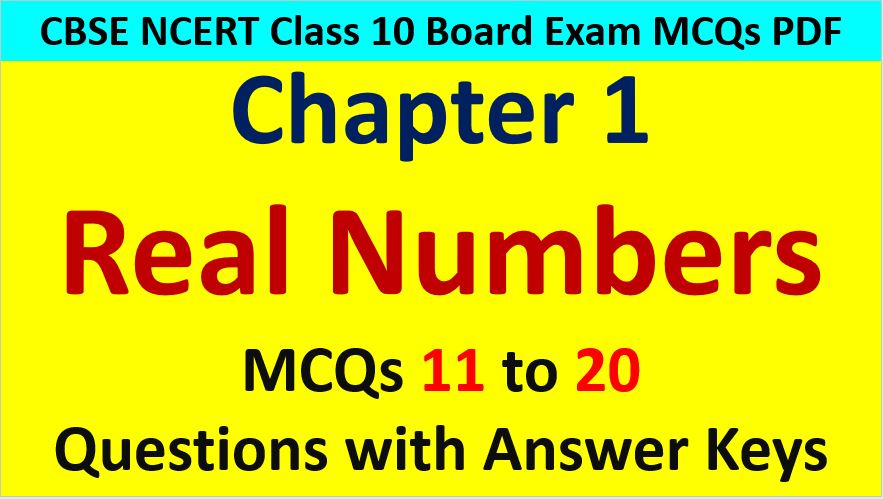Friday, October 22, 2021
Home > CBSE Class 10 > Real Numbers Class 10 Maths MCQ with Answer Keys Solutions PDF DownloadReal Numbers MCQ Questions for Class 10 Question No 11:

The sum of exponents of prime factors in the prime factorization of 196 is

Option A : 3

Option B : 4

Option C : 5

Option D : 2

Option B : 4

Real Numbers MCQ Questions for Class 10 Question No 12:

n2 – 1 is divisible by 8, if n is

Option A : an integer

Option B : a natural number

Option C : an odd integer

Option D : an even integer

Option C : an odd integer

Real Numbers MCQ Questions for Class 10 Question No 13:

Which one of the following is not an irrational number

Option A : 2 + √3

Option B : 2 + √5

Option C : 2 + √3

Option D : 2 + √4

Option D : 2 + 4

Real Numbers MCQ Questions for Class 10 Question No 14:

The total number of factors of prime number is

Option A : 1

Option B : 0

Option C : 2

Option D : 3

Option C : 2

Real Numbers MCQ Questions for Class 10 Question No 15:

If the HCF of 65 and 117 is expressible in the form 65m – 117, then the value of m is

Option A : 4

Option B : 2

Option C : 1

Option D : 3

Option B : 2

Real Numbers MCQ Questions for Class 10 Question No 16:

The product of two irrational numbers is

Option A : Always an irrational numbers

Option B : Always a rational numbers

Option C : Sometimes rational and sometimes irrational numbers

Option D : None of these

Option C : Sometimes rational and sometimes irrational numbers

Real Numbers MCQ Questions for Class 10 Question No 17:

The HCF and the LCM of 12, 21, 15 respectively are

Option A : 3, 140

Option B : 12, 420

Option C : 3, 420

Option D : 420, 3

Option C : 3, 420

Real Numbers MCQ Questions for Class 10 Question No 18:

The largest number which divides 70 and 125, leaving remainders 5 and 8, respectively, is

Option A : 13

Option B : 65

Option C : 875

Option D : 1750

Option A : 13

Real Numbers MCQ Questions for Class 10 Question No 19:

The number 1.12112111211112111112… is a/an

Option A : An irrational number

Option B : A rational number

Option C : Rational or Irrational number

Option D : None of these

Option A : An irrational number

Real Numbers MCQ Questions for Class 10 Question No 20:

The decimal representation of 11/(23 x 5) will

Option A : terminate after 1 decimal places

Option B : terminate after 2 decimal places

Option C : terminate after 3 decimal places

Option D : not terminate